# Overview: Syllogism Notes | Study UPSC CSAT Preparation - UPSC

## UPSC: Overview: Syllogism Notes | Study UPSC CSAT Preparation - UPSC

The document Overview: Syllogism Notes | Study UPSC CSAT Preparation - UPSC is a part of the UPSC Course UPSC CSAT Preparation.
All you need of UPSC at this link: UPSC

What is Syllogism?

A syllogism is a form of reasoning in which a conclusion is drawn from two or three given propositions or statements. It uses deductive reasoning rather than inductive reasoning.

You have to take the given statements to be true, even if they are at variance from established facts.
Let us see an example of deductive reasoning:
Statements:
(a) All cats are dogs.
(b) All dogs are birds.
Conclusion: All cats are birds.
This conclusion is quite visible. But to solve complex problems we have some standard methods.

Method 1: Analytical Method
Following are the four major types of statements generally asked: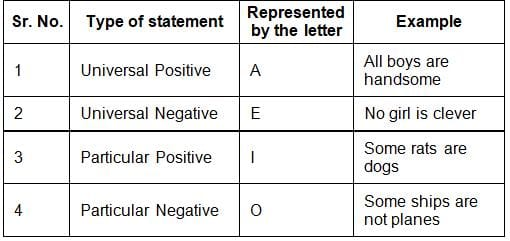While deriving conclusions, the following points should be kept in mind:

• With two particular statements, no universal conclusion is possible.
• With two positive statements, no negative conclusion is possible.
• With two negative statements, no positive conclusion is possible.
• With two particular statements, no conclusion is possible, except when an 'I' type of statement is given and then by reversing it, an 'I' type of conclusion is given.

Important points related to conclusions drawn from single statements:

• A statement of type 'E' when reversed, gives a conclusion of type 'E & O'.
• A statement of type 'A' when reversed, gives a conclusion of type 'I'.
• A statement of type 'I' when reversed, gives a conclusion of type 'I.'
• A statement of type 'O' when reversed, does not give a conclusion of any type.

Method 2: Venn Diagrams

• Another method of solving such types of questions is by drawing a Venn diagram representing the statements.
• However, it is important that all possible Venn diagrams be drawn.
• If a conclusion can be deduced from all the possible solutions, then that conclusion is true.
• If the conclusion can be concluded from one of the possible Venn diagrams and not from the other possible Venn diagram, then that conclusion is taken as false.

Solved Examples

Example 1: Which of the two conclusions can be concluded on the basis of given statements?
Statements:

• Some parrots are scissors.
• Some scissors are not combs.

Conclusions:
I. Some scissors are parrots.
II. Some combs are parrots.

Now, in this case, the possible conclusion is: Some scissors are parrots (I to I), as the universal principle no. 4 says, that with two particular statements only I to I is possible. Therefore, only 1 conclusion is possible. Nothing else is possible.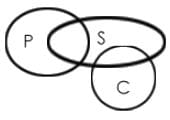Venn diagram

Try yourself:Statements:
Some actors are singers.
- All the singers are dancers.
Conclusions:
1. Some actors are dancers.
2. No singer is an actor.

Example 2: Which of the two conclusions can be concluded on the basis of given statements?
Statements:

• All flowers are candles.
• All lanterns are candles.

Conclusions:
I. Some flowers are lanterns.
II. Some candles are lanterns.

Three possible diagrams are shown above for the given statements.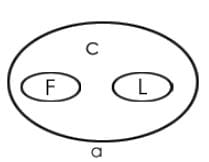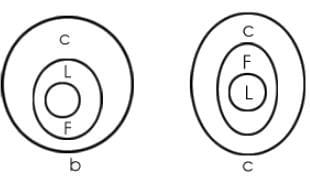Conclusion I follows from the last two possible solutions but does not follow from the first possible solution. Therefore, this conclusion is false.

Conclusion II follows from all the three possible solutions.
Therefore, conclusion II is true.

Try yourself:Statements:
- No door is a dog.
- All the dogs are cats.
Conclusions:
(A) No door is a cat.
(B) No cat is a door.
(C) Some cats are dogs.
(D) All the cats are dogs.

Example 3: Which of the two conclusions can be concluded on the basis of given statements?
Statements:

• All prisoners are men.
• No man is educated.

Conclusions:
I. All prisoners are uneducated.
II. Some men are prisoners.

Two possible diagrams are shown below for the given statements.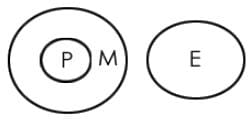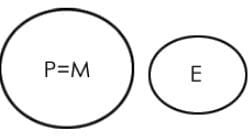Conclusion I follow from both the possibilities, so the conclusion I am true.

Conclusion II also follows from both the possibilities, so conclusion II is also true.

Therefore, both conclusions are true.

Try yourself:Statements:
- All the harmoniums are instruments.
- All the instruments are flutes.
Conclusions:
1. All the flutes are instruments.
2. All the harmoniums are flutes.

Example 4: Which of the two conclusions can be concluded on the basis of given statements?
Statements:

• All sides are lengths.
• No length is a breadth.

Conclusions:
I. All lengths are sides.

Two possible diagrams are shown below for the given statements.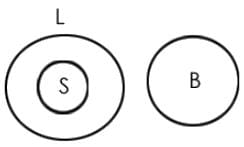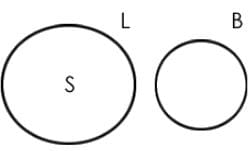Conclusion I: False (conclusion follows from the second possibility but doesn't follow from the first possibility)
Conclusion II: True (conclusion follows from both the Venn diagram possibilities.)

Therefore, only conclusion II is true.

Try yourself:Statements:
Some papers are pens.
- All the pencils are pens.
Conclusions:
1. Some pens are pencils.
2. Some pens are papers.

Example 5:
Statements:

1. All dogs are asses.
2. All asses are bulls.

Conclusions:

1. Some dogs are not bulls.
2. Some bulls are dogs.
3. All bulls are dogs.
4. All dogs are bulls.

On the basis of both statements, the following one diagram is possible.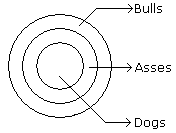From the diagram it is clear that (2) and (4) conclusions logically follow.

Example 6:
Statements:

1. Some dogs are asses.
2. Some asses are bulls.

Conclusions:

1. Some asses are not dogs.
2. Some dogs are bulls.

From these given statements the following diagrams are possible: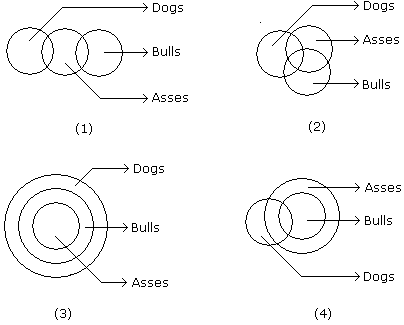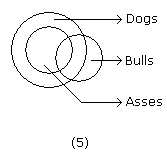From the diagram neither (1) nor (2) conclusions follow.

Directions to Solve: In each of the following questions two statements are given and these statements are followed by two conclusions numbered (1) and (2). You have to take the given two statements to be true even if they seem to be at variance from commonly known facts. Read the conclusions and then decide which of the given conclusions logically follows from the two given statements, disregarding commonly known facts.

• (A) If only (1) conclusion follows
• (B) If sonly (2) conclusion follows
• (C) If either (1) or (2) follows
• (D) If neither (1) nor (2) follows and
• (E) If both (1) and (2) follow.

Example 7:
Statements: Some actors are singers. All the singers are dancers.
Conclusions:

1. Some actors are dancers.
2. No singer is actor.

A. Only (1) conclusion follows
B. Only (2) conclusion follows
C. Either (1) or (2) follows
D. Neither (1) nor (2) follows
E. Both (1) and (2) follow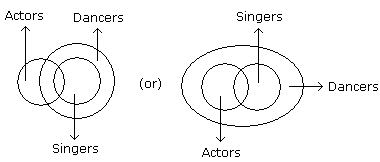Only (1) Follows.

Example 8:
Statements: All the harmoniums are instruments. All the instruments are flutes.
Conclusions:

1. All the flutes are instruments.
2. All the harmoniums are flutes.

A. Only (1) conclusion follows
B. Only (2) conclusion follows
C. Either (1) or (2) follows
D. Neither (1) nor (2) follows
E. Both (1) and (2) follow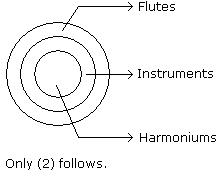Example 9:
Statements: Some mangoes are yellow. Some tixo are mangoes.
Conclusions:

1. Some mangoes are green.
2. Tixo is a yellow.

A. Only (1) conclusion follows
B. Only (2) conclusion follows
C. Either (1) or (2) follows
D. Neither (1) nor (2) follows
E. Both (1) and (2) follow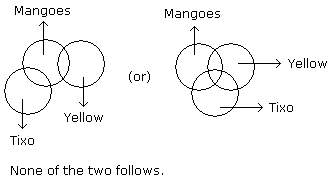The document Overview: Syllogism Notes | Study UPSC CSAT Preparation - UPSC is a part of the UPSC Course UPSC CSAT Preparation.
All you need of UPSC at this link: UPSCUse Code STAYHOME200 and get INR 200 additional OFF

## UPSC CSAT Preparation

58 videos|64 docs|90 tests

### How to Prepare for UPSC

Read our guide to prepare for UPSC which is created by Toppers & the best Teachers

Track your progress, build streaks, highlight & save important lessons and more!

,

,

,

,

,

,

,

,

,

,

,

,

,

,

,

,

,

,

,

,

,

;